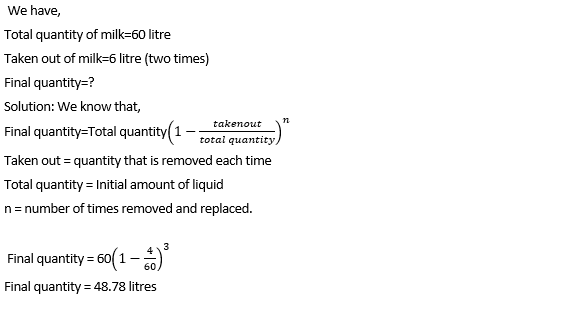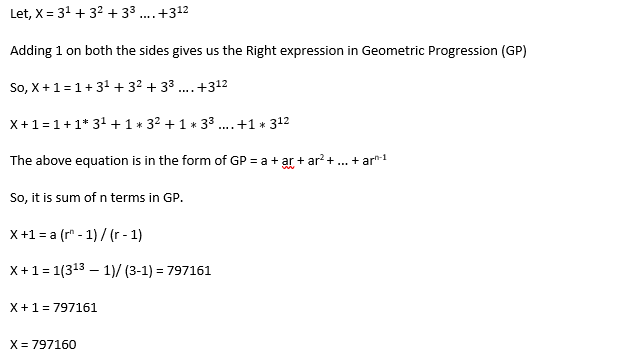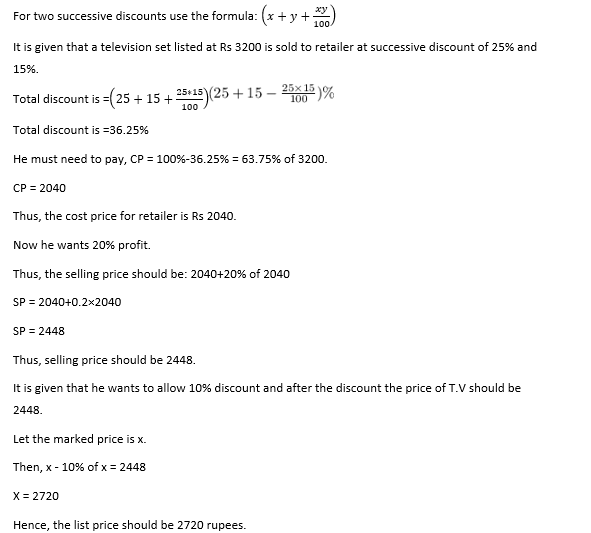# LTI Quantitative Ability Previous Year Questions

28 January 2023

LTI Quantitative Ability Previous Year Questions

LTI Quantitative Ability Previous Year Questions

Q1) In how many ways can 8 letters be posted in 4 post boxes, if any number of letters can be posted in all of the four post boxes?

A. 88

B.  48

C. 68

D. 58

Explanation: Each letter can be posted in any letter box. Each letter has 4 options to get posted.

So, number of ways = 48

Q2) 40% of 80 is x% more than 20% of 120. Find x.

A. 38.33%

B. 35.33 %

C. 39.33%

D. 33.33%

Explanation: 40% of 80 is x% more than 20% of 120

40% of 80 = 32

20% of 120 = 24

32 is x% more than 24

24+x% of 24 = 32

X% of 24 = 8

X = 33.33

Q3) Three years back, a father was 24 years older than his son. At present, the father is 5 times as old as the son. How old will the son be three years from now?

A. 12 years

B. 9 years

C. 15 years

D. 10 years

Explanation: Let Son = x,

father was 24 years older than his son (difference is always same)

father = x+24

at present father is 5 times of son

X+24 = 5x

4x =24

X=6

After three years son will be 6+3 = 9 years

Q4) A number when divided by a divisor leaves a remainder of 25. When twice the original number is divided by the same divisor, the remainder is 11. What Is the value of the divisor?

A. 52

B. 39

C. 26

D. 46

Explanation:

Let number = X, divisor = Y

X = Y*Q + 25

Twice the number

2X = Y*Q1 +11

Sub X

2 (Y*Q + 25) = Y*Q1 +11

2YQ +50 = YQ1 +11

YQ1 – 2YQ = 39

Y(Q1-2Q) = 39

Y = 39 /(Q1-2Q)

Since 39 is a prime number, then(Q1-2Q) = 39 OR 1

Y = 1 or 39

Option B

Q5) A, B and C, each of them working alone can complete a Job in 10, 12 and 15 days respectively. If all three of them work together to complete a job and earn Rs. 6000, what will be C's share of the earnings?

A. Rs 1500

B. Rs 1600

C. Rs 1300

D. Rs 1200

Explanation: Work done is divided as efficiency of them

Efficiency = 1/ time

Ratio of work done = (1/10 ) : (1/12) : (1/15) = 6:5:4

Total amount 6000 will be divided as 6:5:4 , among A,B& C

C’ share = 4/15 * 6000 = 1600/-

Q6) The ratio of two numbers is 7:4 and their H.C.F is 4. Their L.C.M is:

A. 112

B. 116

C. 124

D. 148

Explanation: Let the numbers be 7x and 4x.

Then, their HCF =x

So, x=4

So, the numbers are 7x=28 and 4x=16.

LCM of 28 and 16 is 112.

Q7) A is twice as good as  B and together they finish a piece of work in 28 days. In how many days will A alone finish the work?

A.30 days

B.42 days

C.53 days

D. 40 days

Explanation: Efficiency of A and B are in the ratio of 2: 1

Let efficiency of A be 2 units/day

Let efficiency of B be 1 unit/day

Both of them can complete the task in 28 days

Total work = 3*28 = 84 units

Time taken by A alone = 84/2 = 42 days.

Q8) A container contains 60 liters of milk. From this container, 4 liters of milk was taken out and replaced by water. This process was repeated further two times. How much milk is now contained by the container?

A. 46.34 liters

B. 47.36 liters

C. 48.78 liters

D. 49.16 liters
Explanation:Q9) At what rate percent per annum will a sum of money double in 6 years?

A.(53/3)% p.a

B.(50/3)% p.a

C.(57/3)% p.a

D. (56/3)% p.a

Explanation:
Let P=x, then A=2x
Also, S.I = A−P = 2x−x = x
T = 6 years
We know that S.I. = (P×R×T)/100?)
(x×R×6)/100 = x
R = 100x/6x ?= 16.6% = 50/3%

Q10) Find the lowest common multiple of 1/3, 5/6, 2/9, 4/27

A.1/54

B.1/36

C.20/3

D. 10/27

Explanation:Q11) Type A, 12 kg of rice worth Rs. 40/kg is mixed with Type B, rice worth Rs. 24/kg. What should be the quantity of Type B rice, if the mixture is sold at Rs. 45/kg with 25% profit added in it?

A. 18 Kg

B. 48 Kg

C. 4 Kg

D. Can't say

Explanation: Let, ‘x’ kg if type - B rice should be added in the mixture.

Now, 40*12 + 24*x = 45*100/125*(12+x)

480 + 24x = 36x + 432

12x = 480 - 432 = 48

x = 48/12 = 4

So, the answer is 4 kg.

Q12) A person spends 1/7th of his salary on travel,1/3rd of the remaining on food, he then spends 1/4th of the remaining on rent. Finally he puts 1/6th of the remaining as a monthly savings, after which he has 25000 left. What is his salary (in Rs.)?

A. 70,000

B. 14,000

C. 84,000

D. 26,000

Explanation: Travel = 1/7

Remaining = 7/7 - 1/7 = 6/7

Food = 1/3 × 6/7 = 2/7

Remaining = 6/7 - 2/7  = 4/7

Rent = 1/4 × 4/7 = 1/7

Remaining = 4/7 - 1/7 = 3/7

Savings = 1/6 × 3/7 = 1/14

Remaining = 3/7 - 1/14 = 5/14

5/14 = 25000

Salary = 14/5 × 25000 = Rs. 70000

Q13) 3+32 +33 +.........+312 =?

A. 792044

B. 791022

C. 797160

D. 794028

Explanation:Q14) A TV set listed at Rs 3200 is sold to a retailer at a successive discount of 25% and 15%. The retailer desires a profit of 20%, after allowing a discount of 10% to the customer. At what price should he list the TV set (in Rs.)?

A. 2720

B. 2448

C. 2040

D. 2133

Explanation:Q15) What is the smallest 4-digit number that is divisible by 12, 13 and 15?

A.1990

B.1560

C.2100

D. 1150

Explanation: The smallest number that is divisible by 12, 13 and 15 can be derived from = LCM of (12, 13, 15)

LCM = 780, which is a 3-digit number.

Since the answer should be a 4-digit number.

We can take the 2nd multiple of 780, which is 1560 and that is the smallest 4-digit number that is divisible by 12, 13 and 15.

##